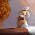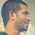VHDL coding tips and tricks: 3 : 8 Decoder using basic logic gates

## Sunday, March 28, 2010

### 3 : 8 Decoder using basic logic gates

Here is the code for 3 : 8 Decoder using basic logic gates such as AND,NOT,OR etc.The module has one 3-bit input which is decoded as a 8-bit output.

--libraries to be used are specified here
library IEEE;
use IEEE.STD_LOGIC_1164.ALL;

--entity declaration with port definitions
entity decoder is
port(    input :        in std_logic_vector(2 downto 0);  --3 bit input
output : out std_logic_vector(7 downto 0)  -- 8 bit ouput
);
end decoder;
--architecture of entity
architecture Behavioral of decoder is

begin
output(0) <= (not input(2)) and (not input(1)) and (not input(0));
output(1) <= (not input(2)) and (not input(1)) and input(0);
output(2) <= (not input(2)) and input(1) and (not input(0));
output(3) <= (not input(2)) and input(1) and input(0);
output(4) <= input(2) and (not input(1)) and (not input(0));
output(5) <= input(2) and (not input(1)) and input(0);
output(6) <= input(2) and input(1) and (not input(0));
output(7) <= input(2) and input(1) and input(0);

end Behavioral;

The test bench program used for testing the design is given below:

library IEEE;
use IEEE.STD_LOGIC_1164.ALL;

--this is how entity for your test bench code has to be declared.
entity testbench is
end testbench;

architecture behavior of testbench is
--signal declarations.
signal input : std_logic_vector(2 downto 0) :=(others => '0');
signal output :  std_logic_vector(7 downto 0) :=(others => '0');

begin
--entity instantiation
UUT : entity work.decoder port map(input,output);

--definition of simulation process
tb : process
begin
input<="000";  --input = 0.
wait for 2 ns;
input<="001";   --input = 1.
wait for 2 ns;
input<="010";   --input = 2.
wait for 2 ns;
input<="011";   --input = 3.
wait for 2 ns;
input<="100";   --input = 4.
wait for 2 ns;
input<="101";   --input = 5.
wait for 2 ns;
input<="110";   --input = 6.
wait for 2 ns;
input<="111";   --input = 7.
wait;
end process tb;

end;

The simulated waveform is shown below:
The code was synthesized using XILINX ISE XST . The RTL schematic of the design is shown below:
Note :- Use RTL Viewer to get a closer look on how your design is implemented in hardware.

#### 5 comments:

1.2.can u plz give me a code for a 32 * 32 bit ram
my email id is smrutisoumya.mishra@gmail.com
the ram must hav 4 output ports each of 8 bit size

3.Can u plz explain me one program in detail. ........

4.i need additive white gaussian noise vhdl code..plz help me

5.You can find some logic gate templates in creately diagram community.